# Central Science: Chapter 19 Flashcards

Set Details Share
created 3 years ago by Chemistry4Life
3,125 views
updated 3 years ago by Chemistry4Life
College: First year, College: Second year, College: Third year, College: Fourth year
Subjects:
chemistry
Page to share:
Embed this setcancel
COPY
code changes based on your size selection
Size:
X

1

The first law of thermodynamics can be given as ________.
A) ΔE = q + w
B) ΔH°rxn = ΣnΔH°f(products) - ΣmΔH°f(reactants)
C) for any spontaneous process, the entropy of the universe increases
D) the entropy of a pure crystalline substance at absolute zero is zero
E) ΔS = qrev/T at constant temperature

A

2

A reaction that is spontaneous as written ________.
A) is very rapid
B) will proceed without outside intervention
C) is also spontaneous in the reverse direction
D) has an equilibrium position that lies far to the left
E) is very slow

B

3

Of the following, only ________ is not a state function.
A) S
B) H
C) q
D) E
E) T

C

4

When a system is at equilibrium, ________.
A) the reverse process is spontaneous but the forward process is not
B) the forward and the reverse processes are both spontaneous
C) the forward process is spontaneous but the reverse process is not
D) the process is not spontaneous in either direction
E) both forward and reverse processes have stopped

D

5

A reversible process is one that ________.
A) can be reversed with no net change in either system or surroundings
B) happens spontaneously
C) is spontaneous in both directions
D) must be carried out at low temperature
E) must be carried out at high temperature

A

6

Which of the following statements is true?
A) Processes that are spontaneous in one direction are spontaneous in the opposite direction.
B) Processes are spontaneous because they occur at an observable rate.
C) Spontaneity can depend on the temperature.
D) All of the statements are true.

C

7

The thermodynamic quantity that expresses the extent of randomness in a system is ________.
A) enthalpy
B) internal energy
C) bond energy
D) entropy
E) heat flow

D

8

For an isothermal process, ΔS = ________.
A) q
B) qrev/T
C) qrev
D) Tqrev
E) q + w

B

9

Which one of the following is always positive when a spontaneous process occurs?
A) ΔSsystem
B) ΔSsurroundings
C) ΔSuniverse
D) ΔHuniverse
E) ΔHsurroundings

C

10

The entropy of the universe is ________.
A) constant
B) continually decreasing
C) continually increasing
D) zero
E) the same as the energy, E

C

11

The second law of thermodynamics states that ________.
A) ΔE = q + w
B) ΔH°rxn = Σ nΔH°f (products) - Σ mΔH°f (reactants)
C) for any spontaneous process, the entropy of the universe increases
D) the entropy of a pure crystalline substance is zero at absolute zero
E) ΔS = qrev/T at constant temperature

C

12

Which of the following statements is false?
A) The change in entropy in a system depends on the initial and final states of the system and the path taken from one state to the other.
B) Any irreversible process results in an overall increase in entropy.
C) The total entropy of the universe increases in any spontaneous process.
D) Entropy increases with the number of microstates of the system.

A

13

Which one of the following processes produces a decrease of the entropy of the system?
A) dissolving sodium chloride in water
B) sublimation of naphthalene
C) dissolving oxygen in water
D) boiling of alcohol
E) explosion of nitroglycerine

C

14

Consider a pure crystalline solid that is heated from absolute zero to a temperature above the boiling point of the liquid. Which of the following processes produces the greatest increase in the entropy of the substance?
A) melting the solid
B) heating the liquid
C) heating the gas
D) heating the solid
E) vaporizing the liquid

E

15

Which one of the following correctly indicates the relationship between the entropy of a system and the number of different arrangements, W, in the system?
A) S = kW
B) S = k/W
C) S = W/k
D) S = k lnW
E) S = Wk

D

16

The entropy change accompanying any process is given by the equation:
A) ΔS = k lnWfinal
B) ΔS = k Wfinal - k Winitial
C) ΔS = k ln(Wfinal / Winitial)
D) ΔS = k final - k initial
E) ΔS = Wfinal - Winitial

C

17

ΔS is positive for the reaction ________.
A) 2H2 (g) + O2 (g) → 2H2O (g)
B) 2NO2 (g) → N2O4 (g)
C) CO2 (g) → CO2 (s)
D) BaF2 (s) → Ba2+ (aq) + 2F- (aq)
E) 2Hg (l) + O2 (g) → 2HgO (s)

D

18

ΔS is positive for the reaction ________.
A) 2NO (g) + O2 (g) → 2NO2 (g)
B) 2N2 (g) + 3H2 (g) → 2NH3 (g)
C) C3H8 (g) + 5 O2 (g) → 3CO2 (g) + 4 H2O (g)
D) Mg (s) + Cl2 (g) → MgCl2 (s)
E) C2H4 (g) + H2 (g) → C2H6 (g)

C

19

ΔS is positive for the reaction ________.
A) CaO (s) + CO2 (g) → CaCO3 (s)
B) N2 (g) + 3H2 (g) → 2NH3 (g)
C) 2SO3 (g) → 2SO2 (g) + O2 (g)
D) Ag+ (aq) + Cl- (aq) → AgCl (s)
E) H2O (l) → H2O (s)

C

20

ΔS is positive for the reaction ________.
A) 2 Ca (s) + O2 (g) → 2 CaO (s)
B) 2 KClO3 (s) → 2KCl (s) + 3 O2 (g)
C) HCl (g) + NH3 (g) → NH4Cl (s)
D) Pb2+ (aq) + 2Cl- (aq) → PbCl2 (s)
E) CO2 (g) → CO2 (s)

B

21

Which reaction produces a decrease in the entropy of the system?
A) CaCO3 (s) → CaO (s) + CO2 (g)
B) 2C (s) + O2 (g) → 2CO (g)
C) CO2 (s) → CO2 (g)
D) 2H2 (g) + O2 (g) → 2H2O (l)
E) H2O (l) → H2O (g)

D

22

A decrease in the entropy of the system is observed for the reaction ________.
A) 4 NH3 (g) + 5 O2 (g) → 4 NO (g) + 6 H2O (g)
B) 2 HgO (s) → 2 Hg (l) + O2 (g)
C) UF6 (s) → U (s) + 3F2 (g)
D) K (s) + 1/2 I2 (g) → KI (s)
E) H2O (s) → H2O (g)

D

23

Which reaction produces an increase in the entropy of the system?
A) Ag+ (aq) + Cl- (aq) → AgCl (s)
B) CO2 (s) → CO2 (g)
C) H2 (g) + Cl2 (g) → 2 HCl (g)
D) N2 (g) + 3 H2 (g) → 2 NH3 (g)
E) H2O (l) → H2O (s)

B

24

Which of the following reactions would have a negative ΔS?
A) NH4Cl (s) → NH3 (g) + HCl (g)
B) PbCl2 (s) → Pb2+ (aq) + 2Cl- (aq)
C) 2C (s) + O2 (g) → 2CO2 (g)
D) 2SO2 (g) + O2 (g) → 2SO3 (g)
E) H2O (l) → H2O (g)

D

25

ΔS is positive for the reaction ________.
A) Pb(NO3)2 (aq) + 2KI(aq) → PbI2 (s) + 2KNO3 (aq)
B) 2H2O (g) → 2H2 (g) + O2 (g)
C) H2O (g) → H2O (s)
D) NO (g) + O2 (g) → NO2 (g)
E) Ag+ (aq) + Cl- (aq) → AgCl (s)

B

26

For an isothermal process, the entropy change of the surroundings is given by the equation:
A) ΔS = qsys T
B) ΔS = -qsys T
C) ΔS = q lnT
D) ΔS = -q lnT
E) ΔS = -qsys / T

E

27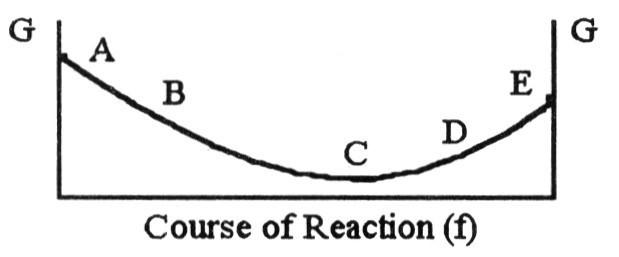The equilibrium position corresponds to which letter on the graph of G vs. f (course of reaction) below?

A) A
B) B
C) C
D) D
E) E

C

28

For the reaction
C2H6 (g) → C2H4 (g) + H2 (g)
ΔH° is +137 kJ/mol and ΔS° is +120 J/K ∙ mol. This reaction is ________.
A) spontaneous at all temperatures
B) spontaneous only at high temperature
C) spontaneous only at low temperature
D) nonspontaneous at all temperatures

B

29

For the reaction
2 C4H10 (g) + 13 O2 (g) → 8 CO2 (g) + 10 H2O (g)
ΔH° is -125 kJ/mol and ΔS° is +253 J/K ∙ mol. This reaction is ________.
A) spontaneous at all temperatures
B) spontaneous only at high temperature
C) spontaneous only at low temperature
D) nonspontaneous at all temperatures

A

30

A reaction that is not spontaneous at low temperature can become spontaneous at high temperature if ΔH is ________ and ΔS is ________.
A) +, +
B) -, -
C) +, -
D) -, +
E) +, 0

A

31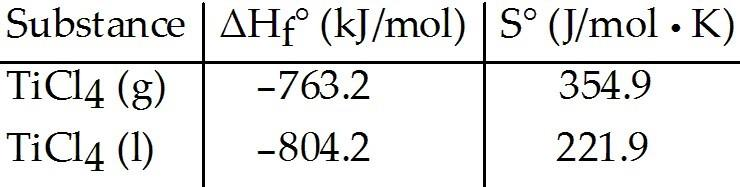Given the following table of thermodynamic data, complete the following sentence. The vaporization of TiCl4 is ________.
A) spontaneous at all temperatures
B) spontaneous at low temperature and nonspontaneous at high temperature
C) nonspontaneous at low temperature and spontaneous at high temperature
D) nonspontaneous at all temperatures
E) not enough information given to draw a conclusion

C

32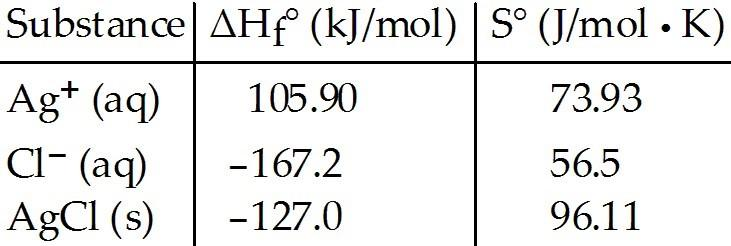Consider the reaction:

Ag+ (aq) + Cl- (aq) → AgCl (s)

Given the following table of thermodynamic data, determine the temperature (in °C) above which the reaction is nonspontaneous under standard conditions.
A) 1230
B) 150
C) 432
D) 133
E) 1640

E

33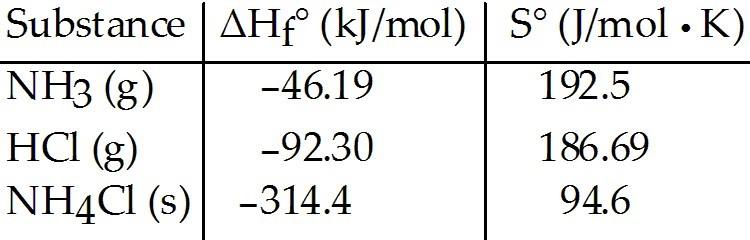Consider the reaction:

NH3 (g) + HCl (g) → NH4Cl (s)
Given the following table of thermodynamic data, determine the temperature (in °C) above which the reaction is nonspontaneous.
A) This reaction is spontaneous at all temperatures.
B) 618.1
C) 432.8
D) 345.0
E) 1235

D

34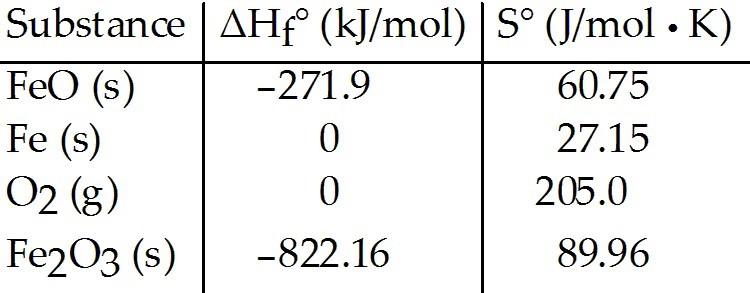Consider the reaction:

FeO (s) + Fe (s) + O2 (g) → Fe2O3 (s)
Given the following table of thermodynamic data, determine the temperature (in °C) at which the reaction is nonspontaneous.
A) below 618.1
B) above 2438
C) above 756.3
D) below 2438
E) This reaction is spontaneous at all temperatures.

B

35

With thermodynamics, one cannot determine ________.
A) the speed of a reaction
B) the direction of a spontaneous reaction
C) the extent of a reaction
D) the value of the equilibrium constant
E) the temperature at which a reaction will be spontaneous

A

36

Which one of the following statements is true about the equilibrium constant for a reaction if ΔG° for the reaction is negative?
A) K = 0
B) K = 1
C) K > 1
D) K < 1

C

37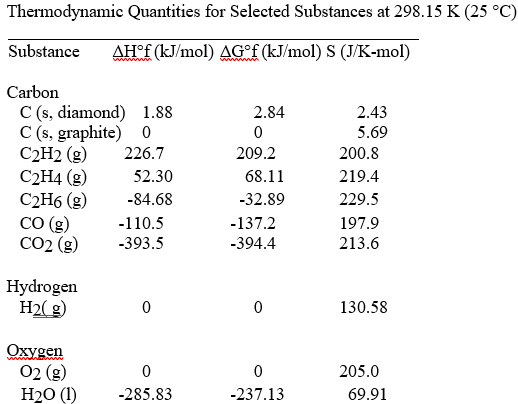The value of ΔS° for the catalytic hydrogenation of acetylene to ethene,

C2H2 (g) + H2 (g) → C2H4 (g)

is ________ J/K∙ mol.
A) +18.6
B) +550.8
C) +112.0
D) -112.0
E) -18.6

D

38The combustion of acetylene in the presence of excess oxygen yields carbon dioxide and water:

2C2H2 (g) + 5O2 (g) → 4CO2 (g) + 2H2O (l)

The value of ΔS° for this reaction is ________ J/K ∙ mol.
A) +689.3
B) +122.3
C) +432.4
D) -122.3
E) -432.4

E

39The value of ΔS° for the reaction

2C (s, diamond) + O2 (g) → 2CO (g)

is ________ J/K ∙ mol.
A) -185.9
B) +185.9
C) -9.5
D) +9.5
E) -195.7

B

40The value of ΔS° for the catalytic hydrogenation of ethene to ethane,

C2H4 (g) + H2(g) → C2H6 (g)

is ________ J/K ∙ mol.
A) -101.9
B) -120.5
C) -232.5
D) +112.0
E) +101.9

B

41The value of ΔS° for the catalytic hydrogenation of acetylene to ethane,

C2H2 (g) + 2H2 (g) → C2H6 (g)

is ________ J/K ∙ mol.
A) -76.0
B) +440.9
C) -232.5
D) +232.5
E) +28.7

C

42The value of ΔS° for the oxidation of carbon to carbon monoxide,

2C (s, graphite) + O2 (g) → 2CO (g)

is ________ J/K ∙ mol. Carbon monoxide is produced in the combustion of carbon with limited oxygen.
A) -12.8
B) +408.6
C) -408.6
D) +179.4
E) +395.8

D

43The value of ΔS° for the oxidation of carbon to carbon dioxide,

C (s, graphite) + O2 (g) → CO2(g)

is ________ J/K ∙ mol. The combustion of carbon, as in charcoal briquettes, in the presence of abundant oxygen produces carbon dioxide.
A) +424.3
B) +205.0
C) -205.0
D) -2.9
E) +2.9

E

44The combustion of ethene in the presence of excess oxygen yields carbon dioxide and water:

C2H4 (g) + 3O2 (g) → 2CO2 (g) + 2H2O (l)

The value of ΔS° for this reaction is ________ J/K ∙ mol.
A) -267.4
B) -140.9
C) -347.6
D) +347.6
E) +140.9

A

45The combustion of ethane in the presence of excess oxygen yields carbon dioxide and water:

2C2H6 (g) + 7O2 (g) → 4CO2 (g) + 6H2O (l)

The value of ΔS° for this reaction is ________ J/K ∙ mol.
A) +718.0
B) -620.1
C) -718.0
D) -151.0
E) +151.0

B

46The combustion of hydrogen in the presence of excess oxygen yields liquid water: What is the value of ΔS° in J/K ∙ mol. for this reaction?
A) -405.5
B) +405.5
C) -265.7
D) -326.3
E) +265.7

D

47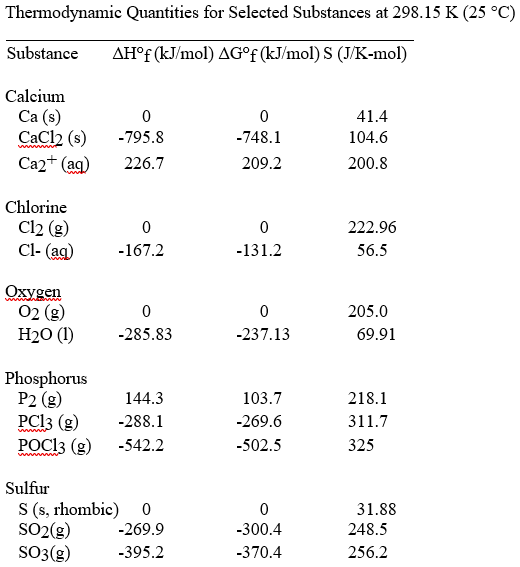The value of ΔS° for the oxidation of solid elemental sulfur to gaseous sulfur trioxide,

2S (s, rhombic) + 3O2(g) → 2SO3 (g)

is ________ J/K ∙ mol.
A) +19.3
B) -19.3
C) +493.1
D) -166.4
E) -493.1

D

48The value of ΔS° for the oxidation of solid elemental sulfur to gaseous sulfur dioxide,

S (s, rhombic) + O2(g) → SO2(g)

is ________ J/K ∙ mol.
A) +485.4
B) +248.5
C) -11.6
D) -248.5
E) +11.6

E

49The value of ΔS° for the decomposition of gaseous sulfur trioxide to solid elemental sulfur and gaseous oxygen,

2SO3 (g) → 2S (s, rhombic) + 3O2 (g)

is ________ J/K ∙ mol.
A) +19.3
B) -19.3
C) +493.1
D) +166.4
E) -493.1

D

50The value of ΔS° for the decomposition of gaseous sulfur dioxide to solid elemental sulfur and gaseous oxygen,

SO2 (g) → S (s, rhombic) + O2 (g)

is ________ J/K ∙ mol.
A) +485.4
B) +248.5
C) -11.6
D) -248.5
E) +11.6

C

51The value of ΔS° for the formation of POCl3 from its constituent elements,

P2 (g) + O2 (g) + 3Cl2 (g) → 2POCl3 (g)

is ________ J/K ∙ mol.
A) -442.0
B) +771.0
C) -321.0
D) -771.0
E) +321.0

A

52The value of ΔS° for the decomposition of POCl3 into its constituent elements,

2POCl3 (g) → P2 (g) + O2 (g) + 3Cl2 (g)

is ________ J/K ∙ mol.
A) +771.0
B) +442.0
C) -321.0
D) -771.0
E) +321.0

B

53The value of ΔS° for the formation of phosphorous trichloride from its constituent elements,

P2 (g) + 3Cl2 (g) → 2PCl3 (g)

is ________ J/K ∙ mol.
A) -311.7
B) +311.7
C) -263.6
D) +129.4
E) -129.4

C

54The value of ΔS° for the decomposition of phosphorous trichloride into its constituent elements,

2PCl3 (g) → P2 (g) + 3Cl2( g)

is ________ J/K ∙ mol.
A) -311.7
B) +311.7
C) +263.6
D) +129.4
E) -129.4

C

55The value of ΔS° for the formation of calcium chloride from its constituent elements,

Ca (s) + Cl2 (g) → CaCl2 (s)

is ________ J/K ∙ mol.
A) -104.6
B) +104.6
C) +369.0
D) -159.8
E) +159.8

D

56The value of ΔS° for the decomposition of calcium chloride into its constituent elements,

CaCl2 (s) → Ca (s) + Cl2 (g)

is ________ J/K ∙ mol.
A) -104.6
B) +104.6
C) +369.0
D) -159.8
E) +159.8

E

57The value of ΔH° for the oxidation of solid elemental sulfur to gaseous sulfur trioxide,

2S (s, rhombic) + 3O2( g) → 2SO3 (g)

is ________ kJ/mol.
A) +790.4
B) -790.4
C) +395.2
D) -395.2
E) +105.1

B

58The value of ΔH° for the decomposition of gaseous sulfur trioxide to its component elements,

2SO3 (g) → 2S (s, rhombic) + 3O2 (g)

is ________ kJ/mol.
A) +790.4
B) -790.4
C) +395.2
D) -395.2
E) +105.1

A

59The value of ΔH° for the oxidation of solid elemental sulfur to gaseous sulfur dioxide,

S (s, rhombic) + O2 (g) → SO2 (g)

is ________ kJ/mol.
A) +269.9
B) -269.9
C) +0.00
D) -11.6
E) +11.6

B

60The value of ΔH° for the decomposition of gaseous sulfur dioxide to solid elemental sulfur and gaseous oxygen,

SO2 (g) → S (s,rhombic) + O2 (g)

is ________ kJ/mol.
A) 0.0
B) +135.0
C) -135.90
D) -269.9
E) +269.9

E

61The value of ΔH° for the formation of POCl3 from its constituent elements,

P2 (g) + O2 (g) + 3Cl2 (g) → 2POCl3 (g)

is ________ kJ/mol.
A) -1228.7
B) -397.7
C) -686.5
D) +1228.7
E) +686.5

A

62The value of ΔH° for the decomposition of POCl3 into its constituent elements,

2POCl3 (g) → P2 (g) + O2 (g) + 3Cl2 (g)

is ________ kJ/mol.
A) -1228.7
B) +1228.7
C) -940.1
D) +940.1
E) 0.00

B

63The value of ΔH° for the formation of phosphorous trichloride from its constituent elements,

P2 (g) + 3Cl2 (g) → 2PCl3 (g)

is ________ kJ/mol
A) -288.1
B) +432.4
C) -720.5
D) +720.5
E) -432.4

C

64The value of ΔH° for the decomposition of phosphorous trichloride into its constituent elements,

2PCl3 (g) → P2 (g) + 3Cl2 (g)

is ________ kJ/mol.
A) +576.2
B) -288.1
C) +720.5
D) +288.1
E) -720.5

C

65The value of ΔH° for the formation of calcium chloride from its constituent elements,

Ca (s) + Cl2 (g) → CaCl2 (s)

is ________ kJ/mol.
A) 0.00
B) -397.9
C) +397.9
D) -795.8
E) +795.8

D

66The value of ΔH° for the decomposition of calcium chloride into its constituent elements,

CaCl2 (s) → Ca (s) + Cl2 (g)

is ________ kJ/mol.
A) 0.00
B) -397.9
C) +397.9
D) -795.8
E) +795.8

E

67The value of ΔG° at 25 °C for the oxidation of solid elemental sulfur to gaseous sulfur trioxide,

2S (s, rhombic) + 3O2 (g) → 2SO3 (g)

is ________ kJ/mol.
A) +740.8
B) -370.4
C) +370.4
D) -740.8
E) +185.2

D

68The value of ΔG° at 25 °C for the oxidation of solid elemental sulfur to gaseous sulfur dioxide,

S (s, rhombic) + O2(g) → SO2 (g)

is ________ kJ/mol.
A) +395.2
B) +269.9
C) -269.9
D) +300.4
E) -300.4

E

69The value of ΔG° at 25 °C for the decomposition of gaseous sulfur trioxide to solid elemental sulfur and gaseous oxygen,

2SO3 (g) → 2S (s, rhombic) + 3O2 (g)

is ________ kJ/mol.
A) +740.8
B) -370.4
C) +370.4
D) -740.8
E) +185.2

A

70The value of ΔG° at 25 °C for the decomposition of gaseous sulfur dioxide to solid elemental sulfur and gaseous oxygen,

SO2 (g) → S (s, rhombic) + O2 (g)

is ________ kJ/mol.

A) +395.2
B) +269.9
C) -269.9
D) +300.4
E) -300.4

D

71The value of ΔG° at 25 °C for the formation of POCl3 from its constituent elements,

P2 (g) + O2 (g) + 3Cl2 (g) → 2POCl3 (g)

is ________ kJ/mol.
A) -1108.7
B) +1108.7
C) -606.2
D) +606.2
E) -1,005

A

72The value of ΔG° at 25 °C for the decomposition of POCl3 into its constituent elements,

2POCl3 (g) → P2 (g) + O2 (g) + 3Cl2 (g)

is ________ kJ/mol.
A) -1108.7
B) +1108.7
C) -606.2
D) +606.2
E) -1,005

B

73The value of ΔG° at 25 °C for the formation of phosphorous trichloride from its constituent elements,

P2 (g) + 3Cl2 (g) → 2PCl3 (g)

is ________ kJ/mol.
A) -539.2
B) +539.2
C) -642.9
D) +642.9
E) -373.3

C

74The value of ΔG° at 25 °C for the decomposition of phosphorous trichloride into its constituent elements,

2PCl3 (g) → P2 (g) + 3Cl2 (g)

is ________ kJ/mol.
A) -539.2
B) +539.2
C) -642.9
D) +642.9
E) -373.3

D

75The value of ΔG° at 25 °C for the formation of calcium chloride from its constituent elements,

Ca (s) + Cl2 (g) → CaCl2 (s)

is ________ kJ/mol.
A) -795.8
B) +795.8
C) +763.7
D) +748.1
E) -748.1

E

76The value of ΔG° at 25 °C for the decomposition of calcium chloride into its constituent elements,

CaCl2 (s) → Ca (s) + Cl2 (g)

is ________ kJ/mol.
A) -795.8
B) +795.8
C) +763.7
D) +748.1
E) -748.1

D

77The value of ΔG° at 373 K for the oxidation of solid elemental sulfur to gaseous sulfur dioxide is ________ kJ/mol. At 298 K, ΔH° for this reaction is -269.9 kJ/mol, and ΔS° is +11.6 J/K.
A) -300.4
B) -274.2
C) -4,597
D) +300.4
E) +4,597

B

78The value of ΔG° at 25 °C for the following reaction:

C2H4 (g) + H2 (g) → C2H6 (g)

is ________ kJ/mol. At 298 K, ΔH° for this reaction is -137.5 kJ/mol, and ΔS° is +120.5 J/K.
A) -35800
B) -173.4
C) 35800
D) -101.7
E) -274.2

B

79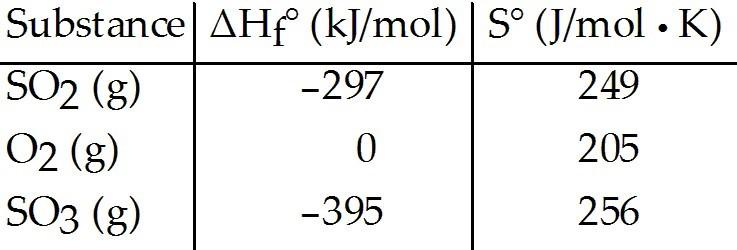Given the thermodynamic data in the table below, calculate the equilibrium constant (at 298 K) for the reaction:
2 SO2 (g) + O2 (g) ⇌ 2 SO3 (g)

A) 2.40 × 1024
B) 1.06
C) 1.95
D) 3.82 × 1023
E) More data are needed.

A

80

The value of ΔG° for a reaction conducted at 25 °C is 3.05 kJ/mol. The equilibrium constant for a reaction is ________ at this temperature.
A) 0.292
B) -4.20
C) 0.320
D) -1.13

A

81

What is the equilibrium constant for a reaction at 25 °C? ΔG° for the reaction is 2.8 kJ/mol.
A) 1.13
B) 0.32
C) -4.2
D) -1.13

B

82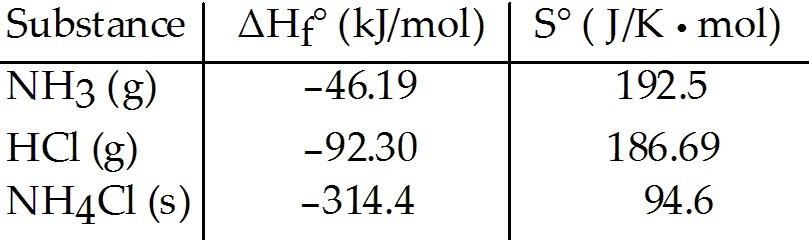Consider the reaction between ammonia and hydrochloric acid to produce ammonium chloride.
Given the following table of thermodynamic data at 298 K:

The value of K for the reaction at 25 °C is ________.
A) 8.4 × 104
B) 150
C) 1.1 × 10-16
D) 9.3 × 1015
E) 1.4 × 108

D

83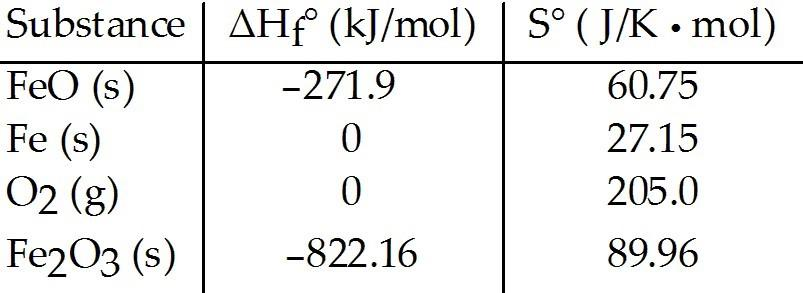Consider the reaction:

FeO (s) + Fe (s) + O2(g) → Fe2O3 (s)
Given the following table of thermodynamic data at 298 K:

The value K for the reaction at 25 °C is ________.
A) 370
B) 5.9 × 104
C) 3.8 × 10-14
D) 7.1 × 1085
E) 8.1 × 1019

D

84

Consider the formation of solid silver chloride from aqueous silver and chloride ions.

Given the following table of thermodynamic data at 298 K:

The value of K for the reaction at 25 °C is ________.
A) 1.9 × 10-10
B) 810
C) 1.8 × 104
D) 3.7 × 1010
E) 5.3 × 109

E

85

The normal boiling point of methanol is 64.7°C and the molar enthalpy of vaporization if 71.8 kJ/mol. The value of ΔS when 1.75 mol of CH3OH(I) vaporizes at 64.7 °C is ________ J/K
A) 0.372
B) 372
C) 1.94 × 103
D) 4.24 × 107
E) 1.94

B

86

The normal boiling point of water is 100.0 °C and its molar enthalpy of vaporization is 40.67 kJ/mol. What is the change in entropy in the system in J/K when 24.7 grams of steam at 1 atm condenses to a liquid at the normal boiling point?
A) 88.8
B) -88.8
C) -150
D) 373
E) -40.7

C

87

The normal boiling point of C2Cl3F3 is 47.6 °C and its molar enthalpy of vaporization is 27.49 kJ/mol. What is the change in entropy in the system in J/K when 28.6 grams of C2Cl3F3 vaporizes to a gas at the normal boiling point?
A) -13.1
B) -4.19
C) 4.19
D) 13.1
E) 27.5

D

88

What is the change in entropy in the system in J/K when 112.2 grams of ethanol at 1 atm condenses to a liquid at the normal boiling point? The normal boiling point of ethanol (C2H5OH) is 78.3 °C and its molar enthalpy of vaporization is 38.56 kJ/mol.
A) 382
B) 1199
C) -267
D) -1199
E) -382

C

89

Which one of the following processes produces a decrease in the entropy of the system?
A) freezing of Fe(l) into Fe(s)
B) evaporation of liquid ethanol into gaseous ethanol
C) dissolution of LiOH(s) in water
D) melting ice to form water
E) mixing of two gases into one container

A

90

ΔS is negative for the reaction ________.
A) Sr(NO3)2 (aq) + 2LiOH (aq) → Sr(OH)2 (s) + 2LiNO3 (aq)
B) 2H2O (g) → 2H2 (g) + O2 (g)
C) CH3OH (l) → CH3OH (g)
D) LiOH (aq) → Li+ (aq) + OH– (aq)
E) C6H12O6 (s) → 6C (s) + 6H2 (g) + 3O2 (g)

A

91

Which of the following has the largest entropy?
A) HI (g)
B) HBr (g)
C) HCl (g)
D) HCl (s)
E) HCl (l)

A

92

Which of the following has the largest entropy?
A) B2F4 (g)
B) BF3 (g)
C) B2H6 (g)
D) BCl3 (l)
E) B2O3 (s)

A

93

Which of the following has the largest entropy at 25 °C and atm?
A) C3H4
B) C3H6
C) C3H8
D) H2
E) C2H6

C

94

Which of the following has the largest entropy at 25 °C and atm?
A) Cl2
B) F2
C) I2
D) Br2
E) O3

C

95

The standard Gibbs free energy of formation of ________ is zero.

(a) H2O (l)
(b) O (g)
(c) CL2 (g)

A) (a) only
B) (b) only
C) (c) only
D) (b) and (c)
E) (a), (b), and (c)

C

96

The standard Gibbs free energy of formation of ________ is zero.

(a)H2 O (l)
(b) Fe (s)
(c) I2 (s)

A) (a) only
B) (b) only
C) (c) only
D) (b) and (c)
E) (a), (b), and (c)

D

97

The standard Gibbs free energy of formation of ________ is zero.

(a) Mn (s)
(b) I2 (s)
(c) Cu (s)

A) (a) only
B) (b) only
C) (c) only
D) (b) and (c)
E) (a), (b), and (c)

E

98

The value of ΔG° at 261.0 °C for the formation of phosphorous trichloride from its constituent elements,

P2(g) +3CL2 (g) → 2PCL3(g)

is ________ kJ/mol. At 25.0 °C for this reaction, ΔH° is -720.5 kJ/mol, ΔG° is -643.9 kJ/mol, and ΔS° is -263.7 J/K
A) -579.6
B) 6.81 × 104
C) 1.40 × 105
D) -651.7
E) -861.4

A

99

The value of ΔG° at 181.0 °C for the formation of calcium chloride from calcium metal and chlorine gas is ________ kJ/mol. At 25.0 °C for this reaction, ΔH° is -795.8 kJ/mol, ΔG° is -748.1 kJ/mol, and ΔS° is -159.8 J/K
A) 7.18 × 104
B) -868.4
C) -766.9
D) -723.2
E) 2.81 × 104

D

100

The signs of ΔH° and ΔS° must be ________ and ________, respectively, for a reaction to be spontaneous at high temperatures but nonspontaneous at low temperatures.
A) +, –
B) –, +
C) –, –
D) +, +
E) +, 0

D

101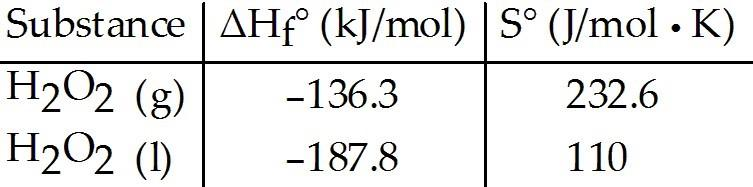Given the following table of thermodynamic data, complete the following sentence. The vaporization of H2O2 (l) is ________.
A) nonspontaneous at low temperature and spontaneous at high temperature
B) spontaneous at low temperature and nonspontaneous at high temperature
C) spontaneous at all temperatures
D) nonspontaneous at all temperatures
E) not enough information given to draw a conclusion

A

102

For the reaction
C(s) + H2O(g) → CO(g) + H2(g)

ΔH° = 133.3 kJ/mol and ΔS° = 121.6 J/K ∙ mol at 298 K. At temperatures greater than ________ °C this reaction is spontaneous under standard conditions.
A) 273
B) 325
C) 552
D) 823
E) 1096

D

103

At what temperature in Kelvin will a reaction have ΔG = 0? ΔH = -24.2 kJ/mol and ΔS = -55.5 J/K-mol and assume both do not vary with temperature.
A) 2.29
B) 2293
C) 298
D) 436
E) 0.436

D

104

At what temperature will a reaction be spontaneous? ΔH = +22.2 kJ/mol and ΔS = +81.1 J/K-mol and assume both do not vary with temperature.
A) at T > 298 K
B) at T < 274 K
C) at T < 298 K
D) at T > 274 K
E) at all temperatures

D

105

For a given reaction, ΔH = +74.6 kJ/mol, and the reaction is spontaneous at temperatures above the crossover temperature, 449 K. The value of ΔS = __________ J/mol ∙ K, assuming that ΔH and ΔS do not vary with temperature.
A) 166
B) 6020
C) -166
D) -6020
E) 3.35 × 104

A

106

For a given reaction, ΔS = +69.0 J/mol∙K, and the reaction is spontaneous at temperatures above the crossover temperature, 439 K. The value of ΔH = __________ kJ/mol, assuming that ΔH and ΔS do not vary with temperature.
A) 30.3
B) -30.3
C) 1.57 × 10-4
D) -1.57 × 10-4
E) 6.36 × 10-3

A

107

For a given reaction with ΔH = -28.1 kJ/mol, the ΔG = 0 at 372 K. The value of ΔS must be __________ J/K-mol, assuming that ΔH and ΔS do not vary with temperature.
A) -75.5
B) 75.5
C) -7.55 × 10-5
D) 7.55 × 10-5
E) -1.32 × 10-2

A

108

For a given reaction with ΔS = -50.8 J/K-mol, the ΔG = 0 at 395 K. The value of ΔH must be __________ kJ/mol, assuming that ΔH and ΔS do not vary with temperature.
A) -20.1
B) 20.1
C) -1.29 × 10-4
D) 1.29 × 10-4
E) -7.78 × 10-3

A

109

What is the equilibrium constant for a reaction at 25 °C. The value of ΔG° is -57.5 kJ/mol.
A) 8.4 × 10101
B) 10
C) 1.0
D) 1.2 × 1010

D

110

If ΔG° for a reaction is less than zero, then ________.
A) K > 1
B) K = 1
C) K < 1
D) K = 0

A

111

In the Haber process, ammonia gas is synthesized from nitrogen gas and hydrogen gas. ΔG° at 298 K for this reaction is -33.3 kJ/mol. The value of ΔG at 298 K for a reaction mixture that consists of 1.9 atm nitrogen gas, 2.3 atm hydrogen gas, and 0.85 atm ammonia gas is ________.
A) -1.0
B) -4.09 × 103
C) -8.62 × 103
D) -118.0
E) -41.9

E

112

Phosphorous and chlorine gases combine to produce phosphorous trichloride. ΔG° at 298 K for this reaction is -642.9 kJ/mol. The value of ΔG at 298 K for a reaction mixture that consists of 1.9 atm P2, 1.6 atm CL2, and PCL3 is ________.
A) -650.1
B) -34.9
C) -714.1
D) -4.46 × 103
E) -7.86 × 103

A

113

The equilibrium constant for a reaction is 0.38 at 25 °C. What is the value of ΔG° (kJ/mol) at this temperature?
A) 2.4
B) -4.2
C) 200
D) 4.2

A

114

The equilibrium constant for the following reaction is 3.0 × 108 at 25 °C.
N2 (g) + 3H2 (g) ⇌ 2NH3 (g)

The value of ΔG° for this reaction is ________ kJ/mol.
A) 22
B) -4.1
C) 4.1
D) -48
E) -22

D

115

A reversible change produces the maximum amount of ________ that can be achieved by the system on the surroundings.

work

116

Calculate ΔG° (in kJ/mol) for the following reaction at 1 atm and 25 °C:

C2H6 (g) + O2 (g) → CO2 (g) + H2O (l) (unbalanced)

ΔGf° C2H6 (g) = -32.89 kJ/mol; ΔGf° CO2 (g) = -394.4 kJ/mol; ΔGf° H2O (l) = -237.13 kJ/mol

-2935.0

117

Calculate ΔG∘ (in kJ/mol) for the following reaction at 1 atm and 25 °C:

C2H6 (g) + O2 (g) → CO2 (g) + H2O (l) (unbalanced)

ΔHf∘ C2H6 (g) = -84.7 kJ/mol; S∘ C2H6 (g) = 229.5 J/K ∙ mol;
ΔHf∘ CO2 (g) = -393.5 kJ/mol; S∘ CO2 (g) = 213.6 J/K ∙ mol;
ΔHf∘ H2O (l) = -285.8 kJ/mol; S∘H2O (l) = 69.9 J/K ∙ mol;
S∘O2 (g) = 205.0 J/K ∙ mol

-2934.0

118

At what temperature (in K) will a reaction become spontaneous? ΔH is 115.0 kJ/mol and ΔS is 75.00 J/K ∙ mol.

1533

119

At what temperature (in K) will a reaction become spontaneous? ΔH is 65.0 kJ/mol and ΔS is 149.00 J/K ∙ mol.

436

120

What is the ΔG° (kJ/mol) for the formation of silver chloride at 25 °C? Ksp = 1.8 × 10-10

56

121

The melting of a substance at its melting point is an isothermal process.

true

122

The vaporization of a substance at its boiling point is an isothermal process.

true

123

The quantity of energy gained by a system equals the quantity of energy gained by its surroundings.

false

124

The entropy of a pure crystalline substance at 0 K is zero.

true

125

The more negative ΔG° is for a given reaction, the larger the value of the corresponding equilibrium constant, K.

true